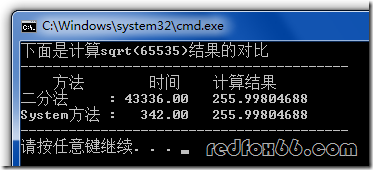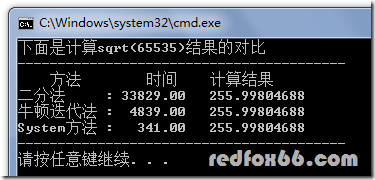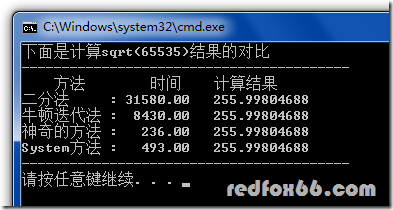# 一个Sqrt函数引发的血案

```float SqrtByBisection(float n) //用二分法
{
if(n<0) //小于0的按照你需要的处理
return n;
float mid,last;
float low,up;
low=0,up=n;
mid=(low+up)/2;
do
{
if(mid*mid>n)
up=mid;
else
low=mid;
last=mid;
mid=(up+low)/2;
}while(abs(mid-last) > eps);//精度控制
return mid;
}```(       4  + 2/4        ) / 2 = 2.25

(     2.25 + 2/2.25     ) / 2 = 1.56944..

( 1.56944..+ 2/1.56944..) / 2 = 1.42189..

( 1.42189..+ 2/1.42189..) / 2 = 1.41423..

```float SqrtByNewton(float x)
{
float val = x;//最终
float last;//保存上一个计算的值
do
{
last = val;
val =(val + x/val) / 2;
}while(abs(val-last) > eps);
return val;
}``````float InvSqrt(float x)
{
float xhalf = 0.5f*x;
int i = *(int*)&x; // get bits for floating VALUE
i = 0x5f375a86- (i>>1); // gives initial guess y0
x = *(float*)&i; // convert bits BACK to float
x = x*(1.5f-xhalf*x*x); // Newton step, repeating increases accuracy
x = x*(1.5f-xhalf*x*x); // Newton step, repeating increases accuracy
x = x*(1.5f-xhalf*x*x); // Newton step, repeating increases accuracy

return 1/x;
}```Quake-III Arena (雷神之锤3)是90年代的经典游戏之一。该系列的游戏不但画面和内容不错，而且即使计算机配置低，也能极其流畅地运行。这要归功于它3D引擎的开发者约翰-卡马克（John Carmack）。事实上早在90年代初DOS时代，只要能在PC上搞个小动画都能让人惊叹一番的时候，John Carmack就推出了石破天惊的Castle Wolfstein, 然后再接再励，doom, doomII, Quake…每次都把3-D技术推到极致。他的3D引擎代码资极度高效，几乎是在压榨PC机的每条运算指令。当初MS的Direct3D也得听取他的意见，修改了不少API。

http://www.fileshack.com/file.x?fid=7547

(下面是官方的下载网址，搜索 “quake3-1.32b-source.zip” 可以找到一大堆中文网页的。ftp://ftp.idsoftware.com/idstuff/source/quake3-1.32b-source.zip)

```float Q_rsqrt( float number )
{
long i;
float x2, y;
const float threehalfs = 1.5F;

x2 = number * 0.5F;
y   = number;
i   = * ( long * ) &y;   // evil floating point bit level hacking
i   = 0x5f3759df - ( i >> 1 ); // what the fuck?
y   = * ( float * ) &i;
y   = y * ( threehalfs - ( x2 * y * y ) ); // 1st iteration
// 2nd iteration, this can be removed
// y   = y * ( threehalfs - ( x2 * y * y ) );

#ifndef Q3_VM
#ifdef __linux__
assert( !isnan(y) ); // bk010122 - FPE?
#endif
#endif
return y;
}```

i = 0x5f3759df – ( i >> 1 );

Lomont为此写下一篇论文，”Fast Inverse Square Root”。 论文下载地址：

http://www.math.purdue.edu/~clomont/Math/Papers/2003/InvSqrt.pdf

http://www.matrix67.com/data/InvSqrt.pdf

```float InvSqrt(float x)
{
float xhalf = 0.5f*x;
int i = *(int*)&x; // get bits for floating VALUE
i = 0x5f375a86- (i>>1); // gives initial guess y0
x = *(float*)&i; // convert bits BACK to float
x = x*(1.5f-xhalf*x*x); // Newton step, repeating increases accuracy
return x;
}```

```float InvSqrt (float x)
{
float xhalf = 0.5f*x;
int i = *(int*)&x;
i = 0x5f3759df - (i>>1);
x = *(float*)&i;
x = x*(1.5f - xhalf*x*x);
return x;
}```

PS. 这个 function 之所以重要，是因为求 开根号倒数 这个动作在 3D 运算 (向量运算的部份) 里面常常会用到，如果你用最原始的 sqrt() 然后再倒数的话，速度比上面的这个版本大概慢了四倍吧… XD

PS2. 在他们追寻的过程中，有人提到一份叫做 MIT HACKMEM 的文件，这是 1970 年代的 MIT 强者们做的一些笔记 (hack memo)，大部份是 algorithm，有些 code 是 PDP-10 asm 写的，另外有少数是 C code (有人整理了一份列表)

TestSqrt.cpp

## 16 条关于 “一个Sqrt函数引发的血案” 的评论

1.Jayuh 留言：

囧！

2. To amorphobia 有main函数~~~

3.china 留言：

惊为天人。。

4.hsy 留言：

请问博主，这个显示函数运行时间（您说是CPU Tick）在C/C++中是怎么调用或是怎么实现的呢？:p

5. 其实在Windows系统中，有很多函数都可以高精度计时：例如，GetTickCount()，QueryPerformanceFrequently()、QueryPerformanceCounter()—本例中用的就是这两个，timeGetSystemTime(),GetCPUTickCount()。
另外还有一个嵌入汇编指令RDTSC也可以高精度计时，你可以到网上查查看。

6.zyk 留言：

在gcc下比系统sqrt快 在vs下sqrt比这个神奇的方法快10倍

•retaw 留言：

看你的回复, 都7102年了. 这个差距估计是你用gcc时候没有加上-O 参数做优化.
现代的x86和x64cpu都内置了一个sqrt指令, 编译器开优化的话, 就是一条汇编, 这种上古优化算法已经没有工程意义了

7.yummy 留言：

%%%，记不住呀

8. 引用通告： LeetCode | 大专栏

9.小天下 留言：

增乘开方法不香吗？只用到加减乘运算，难道不更省运算量？
步骤参考http://k.sina.com.cn/article_2897328623_acb1b9ef01900cfkm.html

10.Young wenkii 留言：

请问有没有matlab开根号版本呀。。。
小白实在不会转化了

11.Visaya Mok 留言：

有意思，抽空用FPGA来看看能不能硬件实现